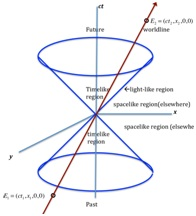# Neutrino Oscillations induced by Space-time

• Category
Science & Technology
• Published
30th Jun, 2021

A study conducted by the National Centre for Basic Sciences (SNBNCBS) shown that the geometry of space-time can cause neutrinos to oscillate.

### Context

A study conducted by the National Centre for Basic Sciences (SNBNCBS) shown that the geometry of space-time can cause neutrinos to oscillate.

• The study was supported under the Department of Science & Technology (DST).

• They are mysterious particles, produced copiously in nuclear reactions in the Sun, stars, and elsewhere.
• Neutrinos interact very weakly.
• They oscillate and different types of neutrinos change into one another.
• The phenomenon of neutrino oscillations requires neutrinos to have tiny masses.
• Probing oscillations of neutrinos and their relations with mass are crucial in studying the origin of the universe.

Findings of the study

• The geometry of space-time can cause neutrino oscillations through quantum effects even if neutrinos are massless.
• Einstein’s theory of general relativity says that gravitation is the manifestation of space-time curvature.
• The neutrinos, electrons, protons, and other particles which are in the category of fermions show a certain peculiarity when they move in presence of gravity.
• Space-time induces a quantum force in addition to gravity between every two fermions.
• This force can depend on the spin of the particles and causes massless neutrinos to appear massive when they pass through matter, like the Sun’s corona or the Earth’s atmosphere.
• Something similar happens for electroweak interactions, and together with the geometrically induced mass, it is enough to cause oscillation of neutrinos.
 Space-time Space-time is a mathematical model which fuses the three dimensions of space and the one dimension of time into a single four-dimensional manifold.  Spacetime diagrams can be used to visualize relativistic effects, such as why different observers perceive differently where and when events occur.